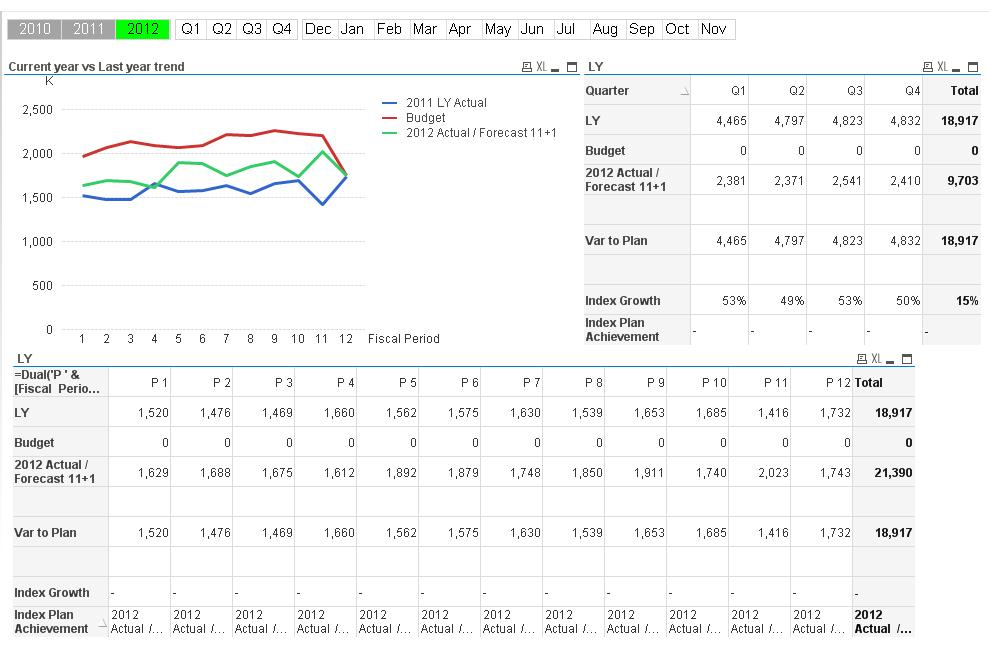# QlikView App Dev

Discussion Board for collaboration related to QlikView App Development.

Announcements
On May 18th at 10AM EDT we will answer your QlikView questions live. REGISTER
cancel
Showing results for
Did you mean:Not applicable

## Dimension not working in summing

Hi I’m working on a new set of charts but I’m facing some silly situation, may be
you can clarify my ideas!!!We want to show sales and forecast data in the same line, but the issue starts when I want to show the data grouping by

So, having the below straight tables…:You can see in the line 2012 Actual / Forecast 11+1, the
table which is showing data by period is ok, but the upper table which is
showing the data by Quarters is not summing each quarter well.

Here is the formula that I’m using.

=if([Fiscal Period]<=cCurrFiscalPeriod,
if(varCurrType = 'L',
Sum(CustProd_SaleAmtLocalCurr)/1000,
Sum(CustProd_SaleAmtUSCurr)/1000

)

,
if(varCurrType = 'L',
Sum(ForecastAmtLocalCurr)/1000,
Sum(ForecastAmtUSCurr)/1000

)

)

In summary, according to my understanding it doesn’t matter
in which way I’m grouping the data (I mean which field I’m using for
dimension). If I’m sum some values, QV should use dimension in order to group
the data and show it accordingly, right??

5 RepliesMVP

If you are using something like

=if([Fiscal Period]<=cCurrFiscalPeriod,

in a dimension context of Quarter, so [Fiscal Period] is more granular and won't return an unambiguous result, the condition will not work as expected by you. You probably need to integrate these kind of conditional statements into your aggregation functions.Not applicable
Author

Thanks for the answer. Any idea about how to do that? I'm a little stuck here!Thanks!MVP

Maybe like this:

=if(varCurrType = 'L',

Sum(

if([Fiscal Period]<=cCurrFiscalPeriod,

CustProd_SaleAmtLocalCurr, ForecastAmtLocalCurr)

)/1000,

Sum(

if([Fiscal Period]<=cCurrFiscalPeriod,

CustProd_SaleAmtUSCurr,ForecastAmtUSCurr)

)/1000

)Not applicable
Author

Thanks for this appoche. Is working almost perfect. The only issue now is regarding the forecast. Running the report now, period 12 should show forecast instead sales, but the amount showed is wrong.

Following your approch, I created this other formula:

=if([Fiscal Year]<max({1}[Fiscal Year]),
Sum(CustProd_SaleAmtUSCurr)/1000,
if([Fiscal Period]<=cCurrFiscalPeriod,
Sum(CustProd_SaleAmtUSCurr)/1000,
Sum({\$<[Fiscal Year]= {\$(=Max([Fiscal Year]))}>} ForecastAmtUSCurr)/1000
))

which work well if I use Fiscal Period as dimension, but again, changing the dimension as Quater, it show Q4 in fiscal Year 2012, but Q1, Q2 and Q3 are hidden.

So, I'm back to the beggining!!!MVP

Well, that's slightly different to my suggested expressions, since you are not embedding the conditional that checks Fiscal Period into the aggregation function.

I am not 100% sure if this is causing the issue or not, without knowing your data model. Could you upload a small sample that demonstrates your issue (could contain some mock-up data, no need for your real business data, but data model should represent your real setting)?